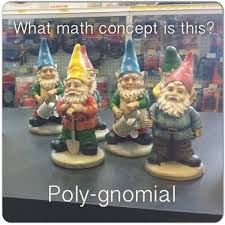Polynomials
3 years ago
mslporter
Save
Edit
Host a game
Live GameLive
Homework
Solo Practice
Practice15 QuestionsShow answers
• Question 1
30 seconds
Q. What is the degree classification of this polynomial?
x²+4x-8
binomial
trinomial
cubic
• Question 2
30 seconds
Q. What is the term classification of the following polynomial?
x³-7x²+3
monomial
binomial
trinomial
polynomial
• Question 3
30 seconds
Q. Which is an example of a linear polynomial?
x²-5
6x+4
5x⁵
2x³-9x²
• Question 4
30 seconds
Q. Which is an example of a cubic binomial?
x³+5
x²+3
x²+5x-6
5x³
• Question 5
30 seconds
Q. What is the leading coefficient of the following polynomial?
5x²-3x+6
2
-3
5
6
• Question 6
30 seconds
Q. Which of these has a negative leading coefficient?
x²+9x-4
6x-9
-8x+8
9x
• Question 7
30 seconds
Q. What is the classification of the following polynomial?
x²-8x+10
cubic trinomial
linear binomial
• Question 8
180 seconds
(x³-2x²+3)+(2x³+3x²-1)
3x³-2x²+3x+2
3x³+x²+2
3x³-5x²-2
3x³-x²+1
• Question 9
180 seconds
(x³-6x²+3)+(3x³+4x-1)
4x³-2x²+2
4x³-6x²+4x+2
-2x³+2x+2
4x³-2x²-2
• Question 10
180 seconds
Q. Subtract the following polynomials:
(3x²-3x+2)-(x²-2x+1)
2x²+x+1
2x²-5x+3
2x²-x+1
4x²-5x+3
• Question 11
180 seconds
Q. Subtract the following polynomials:
(2x³+6x²+4x-2)-(x³+4x²-x)
x³+2x²+5x-2
x³+10x²+3x-2
3x³+2x+4x-3
3x³+10x²-3x-2
• Question 12
30 seconds
Q. What is the GCF in this polynomial?
3x²+6x-18
2
3
6
18
• Question 13
30 seconds
Q. What is the GCF of this polynomial?
2x³-8x²+3x
2
2x
x
• Question 14
30 seconds
Q. What is the GCF of the following polynomial?
4x²+6x-20
1
2
4
6
• Question 15
30 seconds
Q. What is the GCF of the following polynomial?
2x³+4x²-12x?